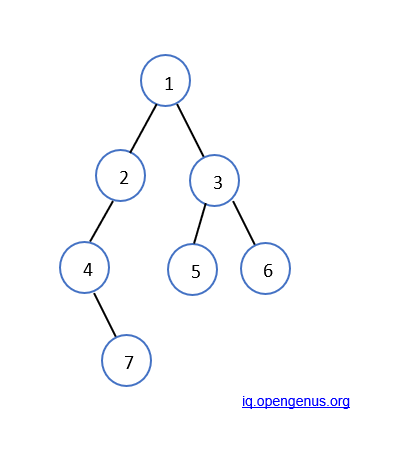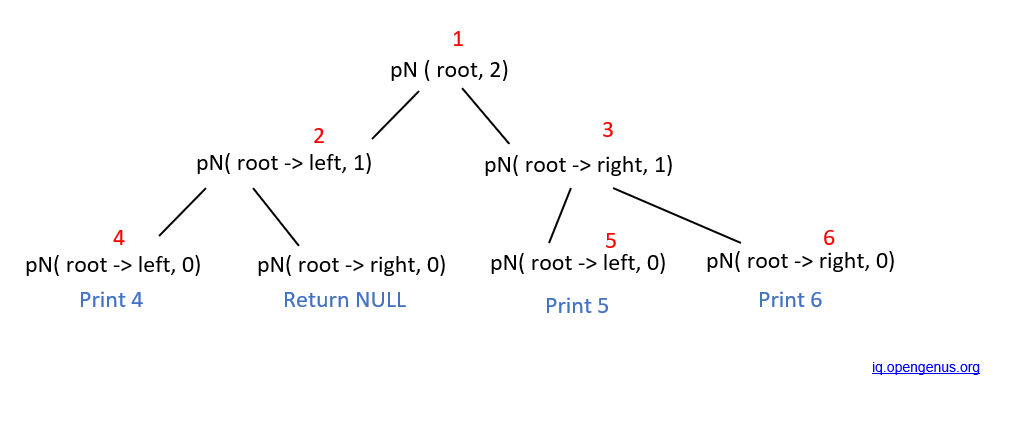×

Search anything:

# Find nodes which are at a distance k from root in a Binary Tree

#### Problems on Binary Tree Algorithms Graph AlgorithmsGet this book -> Problems on Array: For Interviews and Competitive Programming

We are given the root of a tree, and an integer k. We need to print all the nodes which are a distance k from the root.

For example, if the given tree is:Here, the root is 1. If k is 2, then the output should be 4, 5 and 6 as they are at a distance of 2 from the root.

This problem can be solved using a general traversal technique like:

• Depth First Search
• Level Order Traversal

In Depth First Search (DFS) and Breadth First Search (BFS), we can keep track of the level of each node by adding 1 to each level of traversal. When the level is K, the current node is at a distance of K from the root node.

In Level Order traversal, we shall get all nodes at level K.

This problem can be easily solved using general recursion:

• We will make a recursion function, let's say printNodes(node * root, int k) .
• This function will recursively call itself in its left and right children, with a distance of k-1.
• Finally, when k=0 is encountered, we will print the value in the current node. This node will be at a distance of k from the root.

## Walkthrough

Let us walk through the procedure with our given example.

• Initially, the root of the tree is 1 and k = 2. Since k is not equal to 0, we will recursively call the tree with its left child as the root and k-1. Hence, the function printNodes( root->left, 1 ) will be called.
• Our new root will then be 2 and k =1. Again, k is not 0, hence we will call the function printNodes( root->left, 0 ).
• Now, our root is 4 and this time, k=0. So we will print the data in the given root, as this node will be at a distance of k=2 from the original root.
• Similarly, the recursive function will be called in the right subtrees until we get a NULL value, or until k=0.

The following graph depicts the recursion route. (The function name printNodes is abbreviated to pN)## Code

The following is the code to the above problem in C++

``````#include<bits/stdc++.h>

using namespace std;

/* A binary tree node has data,
pointer to left child and
a pointer to right child */
class node
{
public:
int data;
node* left;
node* right;

/* Constructor that allocates a new node with the
given data and NULL left and right pointers. */
node(int data)
{
this->data = data;
this->left = NULL;
this->right = NULL;
}
};

void printNodes(node *root , int k)
{
if(root == NULL)
return;
if( k == 0 )
{
cout << root->data << " ";
return ;
}
else
{
printNodes( root->left, k - 1 ) ;
printNodes( root->right, k - 1 ) ;
}
}

/* Driver code*/
int main()
{

/* Constructed binary tree is
1
/ \
2    3
/    / \
4    5   6
/
7
*/

node *root = new node(1);
root->left = new node(2);
root->right = new node(3);
root->left->left = new node(4);
root->right->left = new node(5);
root->right->right = new node(6);
root->left->left->left = new node(7);

printNodes(root, 2);
return 0;
}

``````

## Output -

``````4 5 6
``````

## Complexity -

Time complexity: O(n)
Space complexity: O(n) , where n is the no. of nodes.

Hence, we have found out how to find nodes at a distance of k from the root node, using recursion.#### Amruta U. Koshe

Amruta is a Computer Science B. Tech student at A. P. Shah Institute of Technology, Thane (2018 to 2022) and has been an Algorithm and Data Structure Developer, Intern at OPENGENUS.

Find nodes which are at a distance k from root in a Binary Tree